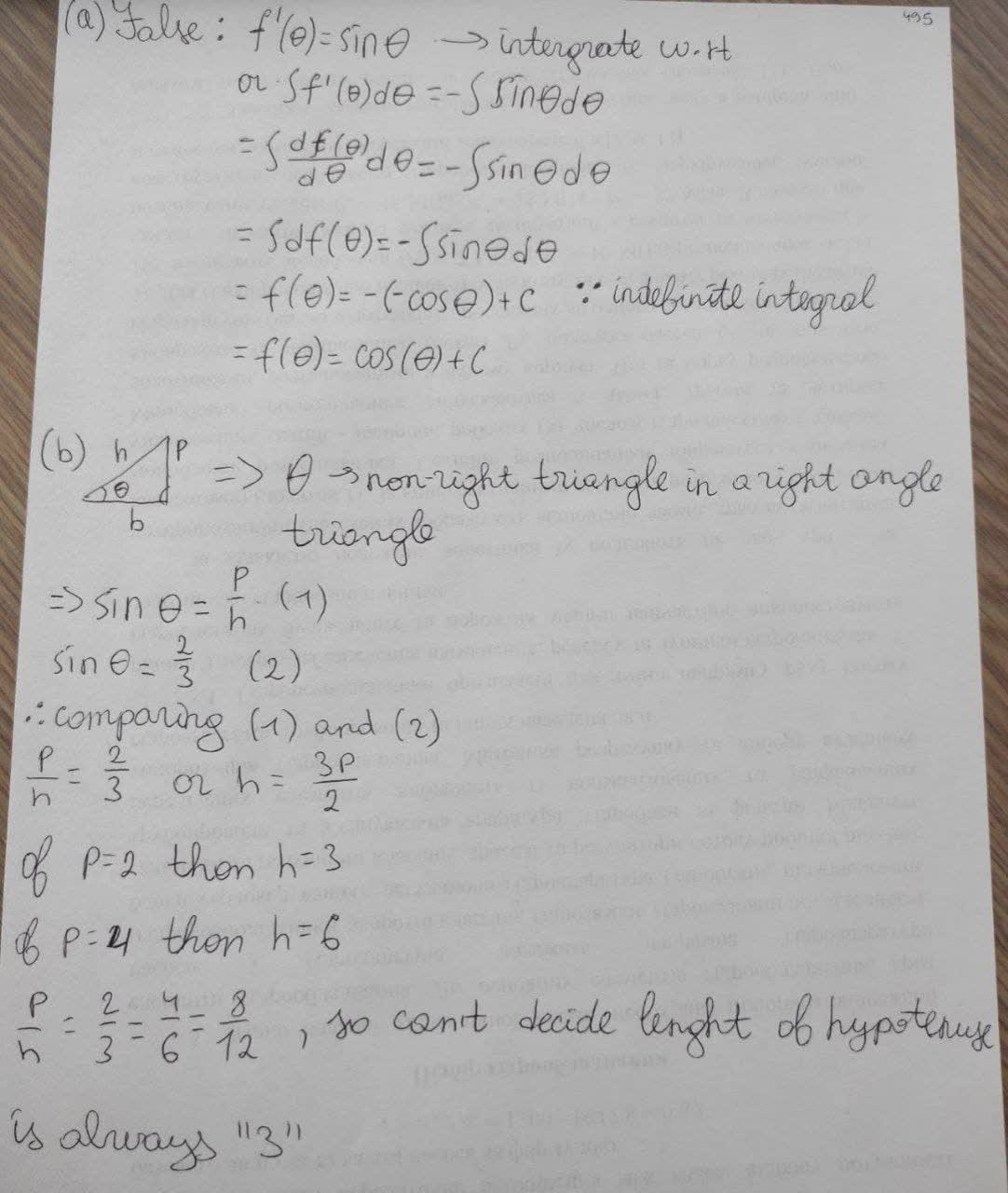# comprehension check for derivatives of trigonometric functions: a) true or false: if fprime(theta) = -sin(theta), then f(theta) = cos(theta). b) trueRivka Thorpe 2020-11-24 Answered

comprehension check for derivatives of trigonometric functions:
a) true or false: if
b) true or false: is one of the non right angles in a right triangle and $\mathrm{sin}\left(\theta \right)=2/3$, then the hypotenuse of the triangle must have length 3.

You can still ask an expert for help

• Questions are typically answered in as fast as 30 minutes

Solve your problem for the price of one coffee

• Math expert for every subject
• Pay only if we can solve itClara Reese
Here is a photo with the answer: